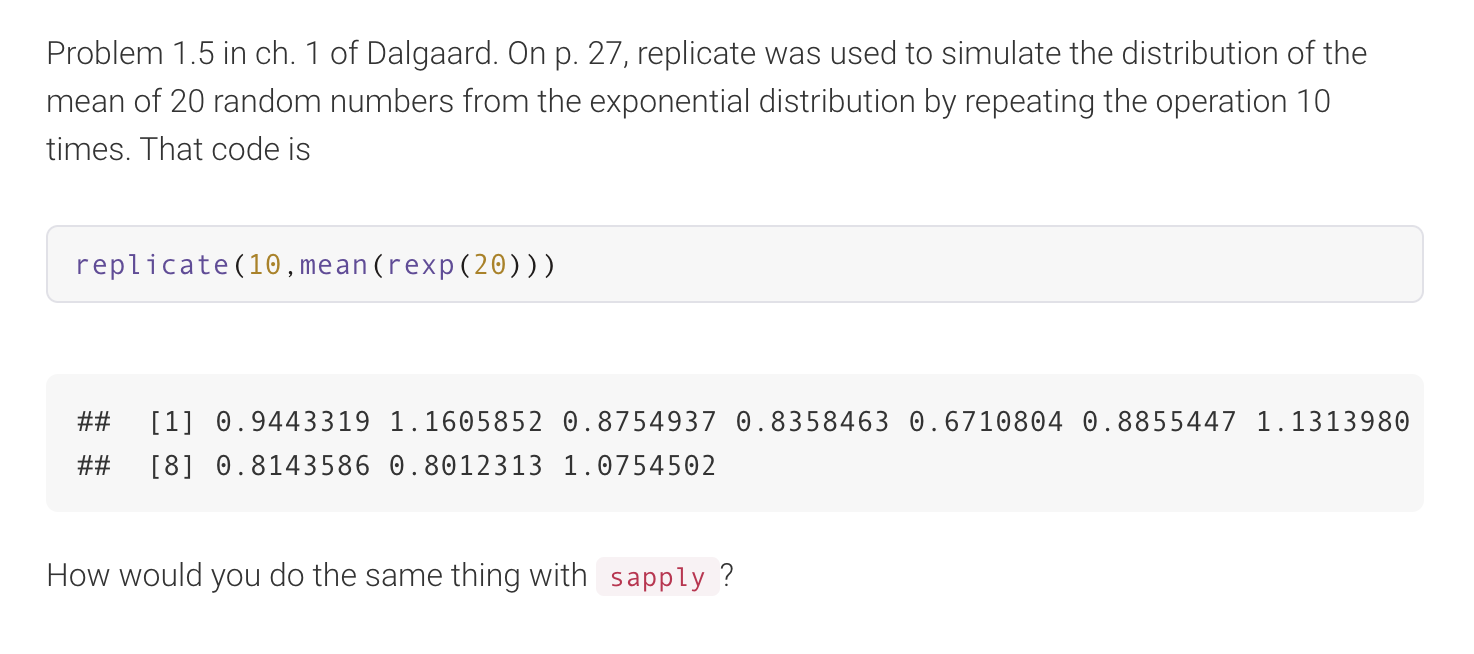Home / Expert Answers / Computer Science / problem-1-5-in-ch-1-of-dalgaard-on-p-27-replicate-was-used-to-simulate-the-distribution-pa390

# (Solved): Problem $$1.5$$ in ch. 1 of Dalgaard. On p. 27, replicate was used to simulate the distribution ...Problem $$1.5$$ in ch. 1 of Dalgaard. On p. 27, replicate was used to simulate the distribution of the mean of 20 random numbers from the exponential distribution by repeating the operation 10 times. That code is \#\#  $$0.8143586 \quad 0.8012313 \quad 1.0754502$$ How would you do the same thing with

We have an Answer from Expert